## 一维数组

``````int main()
{
int arr={0};//创建一个包含有五个元素的整型数组，并将首元素初始化为0
int arr[]={0};//当不确定数组的元素个数时，[]里面可以不用写具体的值
}``````

``````int main()
{
const int n = 10;
int arr[n];//这样来创建一个一维数组是错误的，[]里面必须是一个常量
return 0;
}``````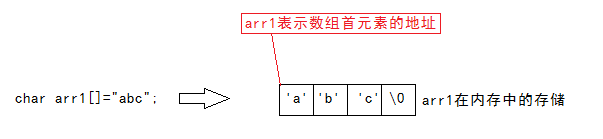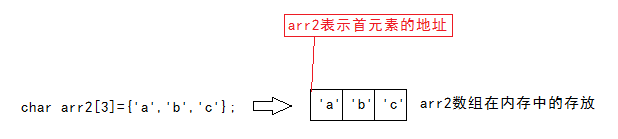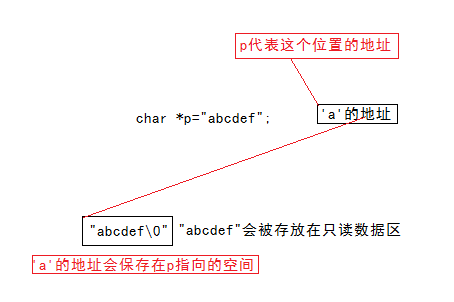``````int main()
{
int i=0;//在内存中，由于i先创建，i一定会存放在高地址处
int arr={0};//相对i位于低地址处
for(i=0;i<=6;i++)
{
arr[i]=0;
}
return 0;
}
//由于数组的越界访问，这个程序会无限的循环下去
//是因为随着数组下标的增长，会越界访问到i所在的空间，继而不断改变i的值``````

VS编译器会在i所在内存与arr所在内存间隔两个内存空间（类型与数组一致）。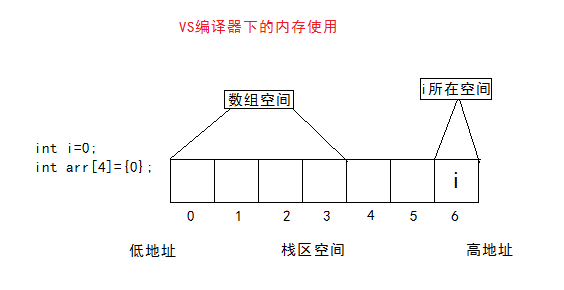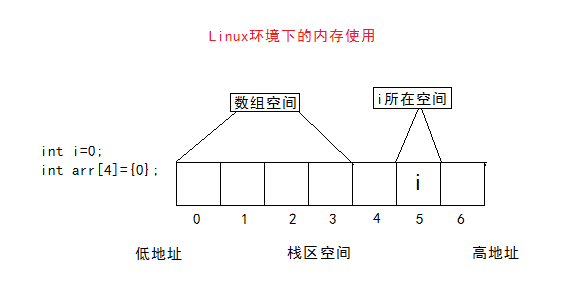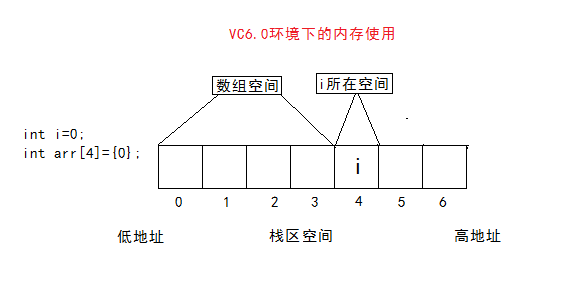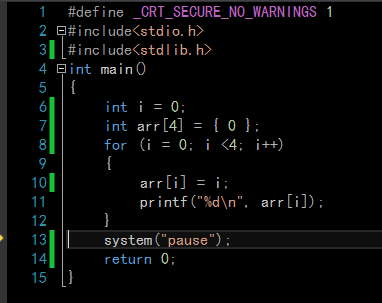&arr: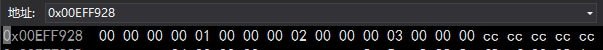&arr: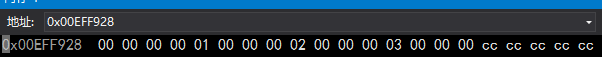&arr: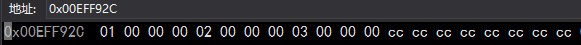&arr: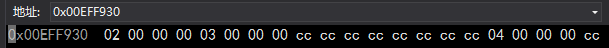&arr: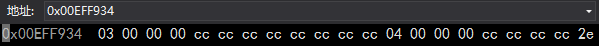``````int arr[] = {0};
int i = 0;
int *p = arr;
*(p+i) = arr[i];
//arr[i]=*(arr+i)=*(p+i)=i[arr]
``````

## 二维数组

``````int main()
{
int arr={0};//3表示行数，4表示列数
int arr1={1,2,3,4,5};//按顺序存放，第一行存1 2 3 4，5存在第二行
int arr2={{1,2,3},{4,5}};//1 2 3存在第一行，4 5存在第二行
int arr3[][常量]={0};//行数可以省略，列数不可以省略
return 0;
]``````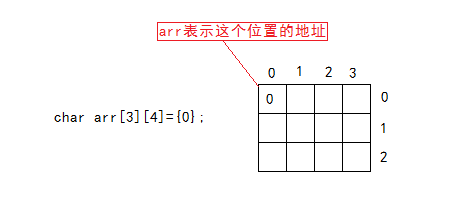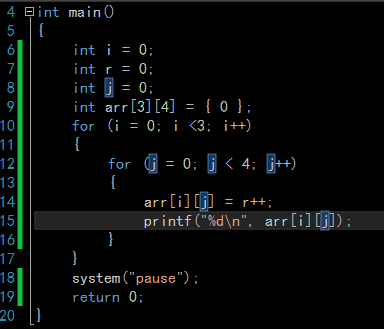&arr: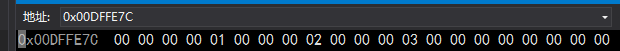&arr: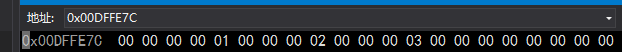&arr: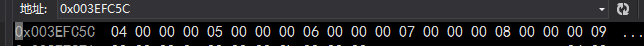&arr: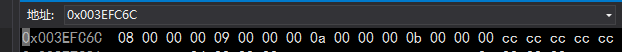``````int main()
{
int arr={0};
return 0;
}``````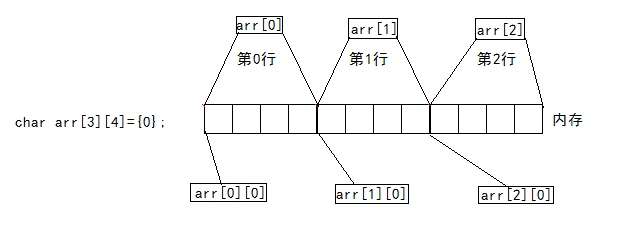## sizeof( )

1.当数组名单独放在sizeof()内时，表示整个数组。
2.&数组名（数组名表示整个数组），取出的是整个数组的地址。